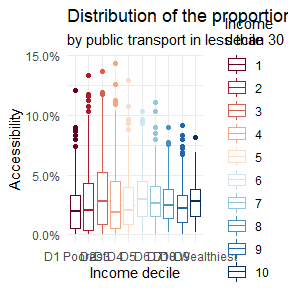2021-04-28

library(aopdata)
library(sf)
#> Warning: package 'sf' was built under R version 4.0.4
library(ggplot2)
library(data.table)
library(units)
#> Warning: package 'units' was built under R version 4.0.4

mode='public_transport',
peak = T,
year=2019,
showProgress = F)

ggplot() +
geom_boxplot(data=subset(df, !is.na(R003)),
aes(x = factor(R003), y=CMAET30, color=factor(R003))) +
scale_color_brewer(palette = 'RdBu') +
labs(title='Distribution of the proportion of jobs accessible', color="Income\ndecile",
subtitle='by public transport in less than 30 min. by income decile',
x='Income decile', y="Accessibility") +
scale_x_discrete(labels=c("D1 Poorest", paste0('D', 2:9), "D10 Wealthiest")) +
scale_y_continuous(labels = scales::percent) +
theme_minimal()Palma ratio

avg_access_10p_wealthiest <- df[ R003==10, weighted.mean(x=CMATT60, w=P001)]
avg_access_40p_poorest <- df[ R003<=4, weighted.mean(x=CMATT60, w=P001)]
palma_ratio <- avg_access_10p_wealthiest / avg_access_40p_poorest

print( paste0('In less than 60 min. by public transport, the 10% wealthiest population could access on average ', round(palma_ratio,2), ' times more job opportunites than the 40% poorest people') )
#>  "In less than 60 min. by public transport, the 10% wealthiest population could access on average 2.65 times more job opportunites than the 40% poorest people"